NSO Result Declared Check here | NSO Level 2 Exam Timing & Guidelines Check here | Register for Maths, Science, English, GK Olympiad Exam Click here | Check Olympiad Exam Dates here | Buy Practice Papers for IMO, IOM, HEO, IOEL etc here | Login here to participate in all India free mock test on every Saturday

# Gravitation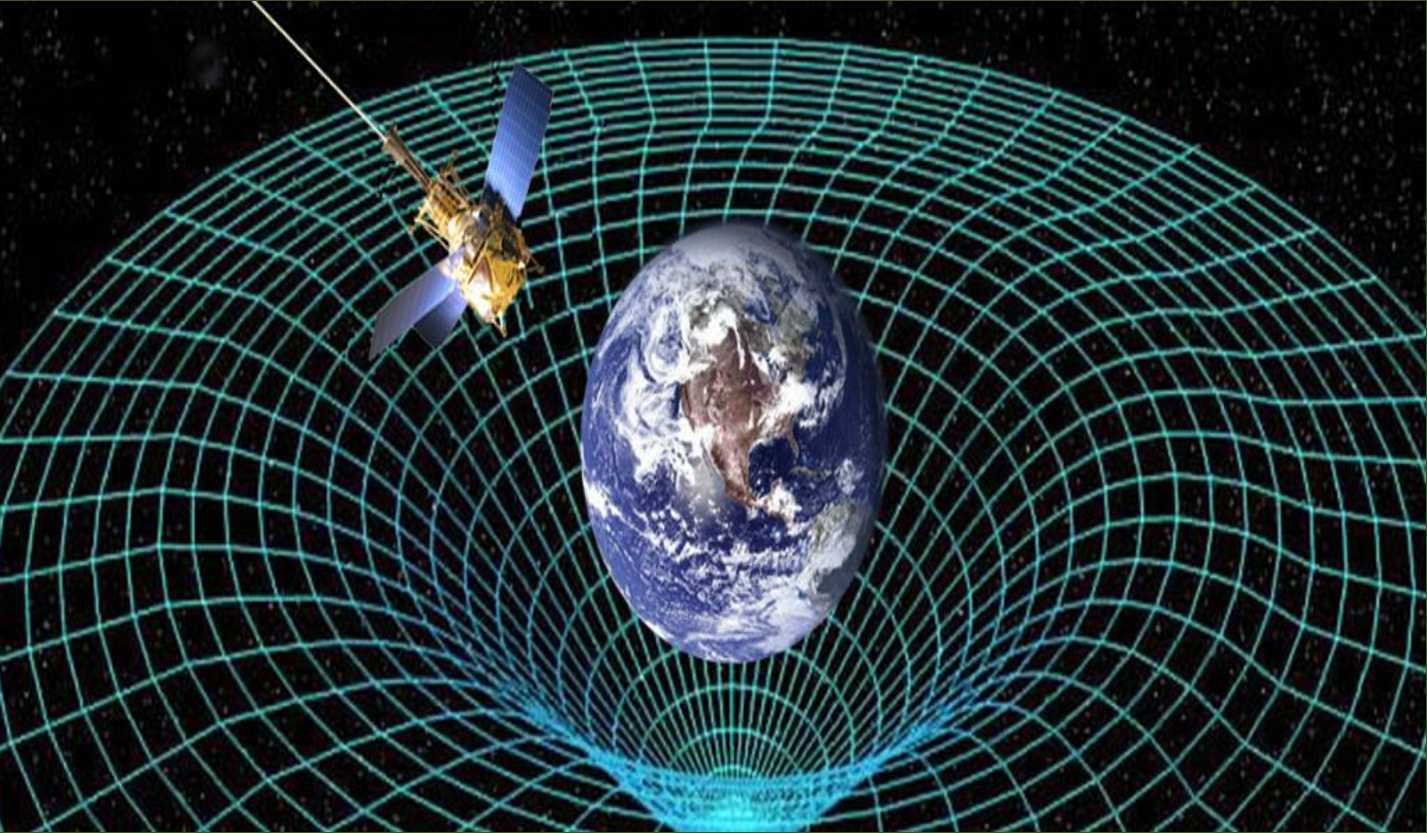Everyone knows that stones fall down from a building. We all know that the planets move around the sun and the moon around the Earth. But do we know the reason why these phenomena exist? This is due to Newton's law of gravitation. This was one of the main discoveries in the world of science and was a revolution in this world.

## Universal Law of Gravitation

Every object in the universe attracts every other object with a force which is proportional to the product of their masses and inversely proportional to the square of the distance between them. The force is along the line joining the centres of two objects.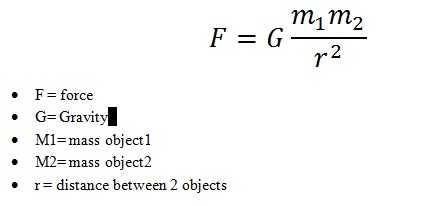#### How Did Newton Find The Inverse Square Law?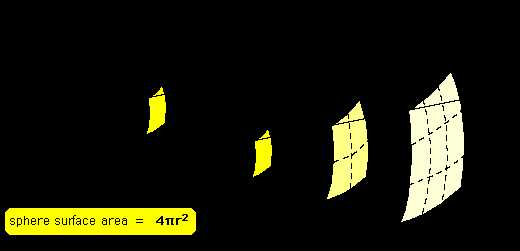There has always been a great interest in the motion of planets. By the 16th century, a lot of data on the motion of planets had been collected by many astronomers. Based on these data, Johannes Kepler derived three laws, which govern the motion of planets.

#### Kepler’s Laws

These are called Kepler’s laws. These are:

• The orbit of a planet is an ellipse with the Sun at one of the foci.
• The line joining the planet and the Sun sweep equal areas in equal intervals of time. Thus, if the time of travel from A to B is the same as that from C to D, then the areas OAB and OCD are equal.
• The cube of the mean distance of a planet from the Sun is proportional to the square of its orbital period T. Or, r3/T2 = constant. It is important to note that Kepler could not give a theory to explain the motion of planets.
• It was Newton who showed that the cause of the planetary motion is the gravitational force that the Sun exerts on them. Newton used the third law of Kepler to calculate the gravitational force of attraction. The gravitational force of the Earth is weakened by distance. A simple argument goes like this.

### Importance of Law of Gravitation

The universal law of gravitation successfully explained several phenomena which were believed to be unconnected:

• The force that binds us to the Earth.
• The motion of the moon around the Earth.
• The motion of planets around the Sun.
• The tides due to the moon and the Sun.

## Mass & Weight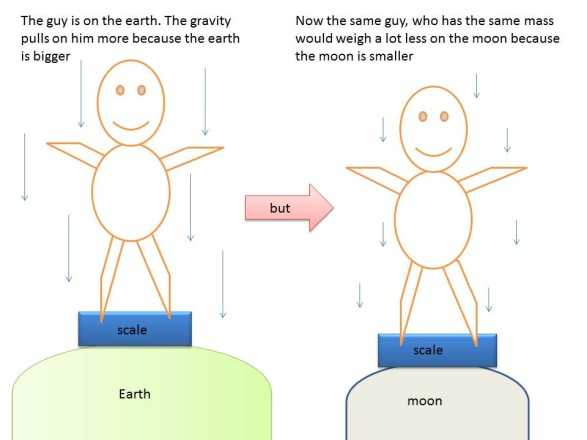Greater the mass, the greater is the inertia. It remains the same whether the object is on the Earth, the moon or even in outer space. Thus, the mass of an object is constant and does not change from place to place.

We know that the Earth attracts every object with a certain force and this force depends on the mass (m) of the object and the acceleration due to the gravity (g). The weight of an object is the force with which it is attracted towards the Earth.

We know that,

that is,

### F = m × g

The force of attraction of the Earth on an object is known as the weight of the object. It is denoted by W.

As the weight of an object is the force with which it is attracted towards the Earth, the SI unit of weight is the same as that of force, that is, Newton (N). The weight is a force acting vertically downwards; it has both magnitude and direction.

## Archimede's Principle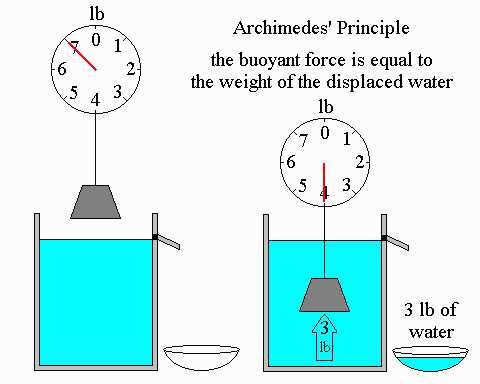We know that the elongation produced in the string or the spring balance is due to the weight of the stone. Since the extension decreases once the stone is lowered in water, it means that some force acts on the stone in an upward direction. Thus, the net force on the string decreases and hence the elongation also decreases. This upward force exerted by water is known as the force of buoyancy. When a body is immersed fully or partially in a fluid, it experiences an upward force that is equal to the weight of the fluid displaced by it.

## Recap

• The law of gravitation states that the force of attraction between any two objects is proportional to the product of their masses and inversely proportional to the square of the distance between them. The law applies to objects anywhere in the universe. Such a law is said to be universal.
• Gravitation is a weak force unless large masses are involved.
• Force of gravitation due to the Earth is called gravity.
• The force of gravity decreases with altitude. It also varies on the surface of the Earth, decreasing from poles to the equator.
• The weight of a body is the force with which the Earth attracts it.
• The weight is equal to the product of mass and acceleration due to gravity.
• The weight may vary from place to place but the mass stays constant.
• All objects experience a force of buoyancy when they are immersed in a fluid.
• Objects having a density less than that of the liquid in which they are immersed, float on the surface of the liquid. If the density of the object is more than the density of the liquid in which it is immersed, then it sinks in the liquid.## Quiz for Gravitation

 Q.1 Pressure is defined as ____________________. a) Force acting per unit volume b) Force acting per unit mass c) Force acting per unit area d) Force which is acting per unit density
 Q.2 The direction of thrust is __________________ to the surface. a) Parallel b) Tangential c) Radial d) Perpendicular
 Q.3 What is the measure used by army tanks to walk on the sand? a) Smaller tyres b) Heavier body c) Smaller chains d) Wide chains around tyres
 Q.4 A sharp knife cuts better than a blunt knife because of __________________. a) Low pressure b) High pressure c) Low volume d) High volume
 Q.5 Mass M is divided into two parts xM and (1 – x)M. For a given separation, the value of x for which the gravitational attraction between the two pieces becomes maximum? a) 1/2 b) 3/5 c) 1 d) 2
 Q.6 The moon's radius is 1/4 that of the earth and its mass is 1/80 times that of the earth. If g represents the acceleration due to gravity on the surface of the earth, that on the surface of the moon will be? a) g/4 b) g/5 c) g/6 d) g/8
 Q.7 If the radius of the earth were to shrink by 1% its mass remaining the same, then what would be acceleration due to gravity on the earth's surface? a) Decrease by 2% b) Increase by 1% c) Remain unchanged d) Increase by 2%
 Q.8 Mass of moon is 7.34 x 1022 kg. If the acceleration due to gravity on the moon is 1.4m/s2, find the radius of the moon? a) 0.56 x 106 b) 1.87 x 106 c) 1.92 x 106 d) 1.01 x 108
 Q.9 A planet has mass 1/10 of that of earth, while radius is 1/3 that of earth. If a person can throw a stone on earth surface to a height of 90m, then at what height he will be able to throw the stone on that planet? a) 90 m b) 40 m c) 100 m d) 45 m
 Q.10 Find the height of the point vertically above the earth's surface, at which acceleration due to gravity becomes 1% of its value at the surface? a) 9R b) 10R c) 20R d) 8R